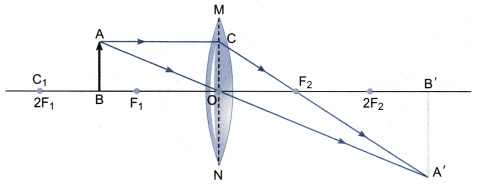# Study the ray diagram given below and answer the following questions

Study the ray diagram given below and answer the following questions1. State the type of lens used in the figure.
2. List two properties of the image formed.
3. In which position of the object will the magnification be -1?